## ML Aggarwal Class 8 Solutions for ICSE Maths Chapter 1 Rational Numbers Ex 1.6

Question 1.
In a bag, there are 20 kg of fruits. If 7$$\frac { 1 }{ 6 }$$ kg of these fruits be oranges and 8$$\frac { 2 }{ 3 }$$ kg of thee are apples and rest are grapes. Find the mass of the grapes in the bag.
Solution:
Total fruits in a bag = 20 kg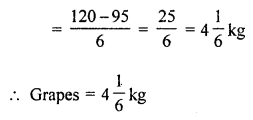Question 2.
The population of a city is 6,63,432. If $$\frac { 1 }{ 2 }$$ of the population are adult males and $$\frac { 1 }{ 3 }$$ of the population are adult females, then find the number of children in the city.
Solution:
Population of a city = 6,63,432
Number of adult males = $$\frac { 1 }{ 2 }$$ of 6,63,432 = 3,31,716
Number of adult females = $$\frac { 1 }{ 3 }$$ of 6,63,432 = 2,21,144
Remaining population = 6,63,432 – (3,31,716 + 2,21,144)
= 6,63,432 – 5,52,860
= 1,10,572
Number of children = 1,10,572

Question 3.
In an election of housing society, there are 30 voters. Each of them gives the vote. Three persons X, Y and Z are standing for the post of Secretary. If Mr X got $$\frac { 2 }{ 5 }$$ of the total votes and Mr Z got $$\frac { 1 }{ 3 }$$ of the total votes, then find the number of votes which Mr Y got.
Solution:
Number of voters = 30
and number of person for election = X, Y, Z
X get $$\frac { 2 }{ 5 }$$ of total votes = $$\frac { 2 }{ 5 }$$ of 30 = 12
Z get $$\frac { 1 }{ 3 }$$ of total votes = $$\frac { 1 }{ 3 }$$ of 30 = 10
Remaining votes = 30 – (12 + 10) = 30 – 22 = 8

Question 4.
A person earns ₹ 100 in a day. If he spent ₹ 14$$\frac { 2 }{ 7 }$$ on food and ₹ 30$$\frac { 2 }{ 3 }$$ on petrol. How much did he save on that day?
Solution:
A man’s earnings per day = ₹ 100Question 5.
In an examination, 400 students appeared. If $$\frac { 2 }{ 3 }$$ of the boys and all 130 girls passed in examination, then find how many boys failed in examination?
Solution:
Number of students appears = 400
Number of boys = $$\frac { 2 }{ 3 }$$ of total boys passed the examination
and all girls 130 passed
Number of total boys = 400 – 130 = 270
Number of students passed = $$\frac { 2 }{ 3 }$$ of 270 = 180
and number of boys failed = 270 – 180 = 90

Question 6.
A car is moving at the speed of 40$$\frac { 2 }{ 3 }$$ km/h. Find how much distance will it cover in $$\frac { 9 }{ 10 }$$ hrs?
Solution:Question 7.
Find the area of a square lawn whose one side is 5$$\frac { 7 }{ 9 }$$ m long.
Solution: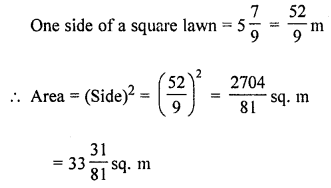Question 8.
Perimeter of a rectangle is 15$$\frac { 3 }{ 7 }$$ m. If the length is 4$$\frac { 2 }{ 7 }$$ m, find its breadth.
Solution:Question 9.
Rahul had a rope of 325$$\frac { 4 }{ 5 }$$ m long. He cut off a 150$$\frac { 3 }{ 5 }$$ m long piece, then he divided the rest of the rope into 3 parts of equal length. Find the length of each part.
Solution:Question 10.
If 3$$\frac { 1 }{ 2 }$$ litre of petrol costs ₹ 270$$\frac { 3 }{ 8 }$$, then find the cost of 4 litre of petrol.
Solution: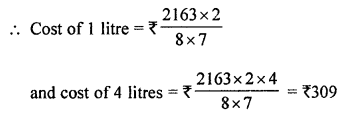Question 11.
Ramesh earns ₹ 40,000 per month. He spends $$\frac { 3 }{ 8 }$$ of the income on food, $$\frac { 1 }{ 5 }$$ of the remaining on LIC premium and then $$\frac { 1 }{ 2 }$$ of the remaining on other expenses. Find how much money is left with him?
Solution:
Monthly earnings of Ramesh = ₹ 40000
Expenditure on food = $$\frac { 3 }{ 8 }$$ of ₹ 40000 = ₹ 15000
Remaining income = 40000 – 15000 = ₹ 25000
Expenditure on LIC premium = $$\frac { 1 }{ 5 }$$ of ₹ 25000 = ₹ 5000
Remaining amount = ₹ 25000 – ₹ 5000 = ₹ 20000
Expenditure on other expenses = $$\frac { 1 }{ 2 }$$ of ₹ 20000 = ₹ 10000
Remaining amount left = ₹ 20000 – ₹ 10000 = ₹ 10000

Question 12.
A, B, C, D and E went to a restaurant for dinner. A paid $$\frac { 1 }{ 2 }$$ of the bill, B paid $$\frac { 1 }{ 5 }$$ of the bill and rest of the bill was shared equally by C, D and E. What fraction of the bill was paid by each?
Solution:
Let total bill of the restaurant = 1
A pays the bill = $$\frac { 1 }{ 2 }$$
B pays the bill = $$\frac { 1 }{ 5 }$$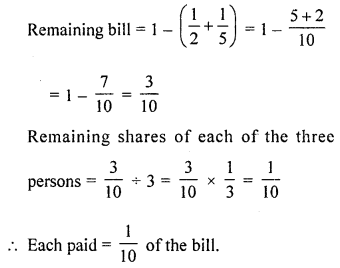Question 13.
$$\frac { 2 }{ 5 }$$ of total number of students of a school come by car while $$\frac { 1 }{ 4 }$$ of students come by bus to school. All the other students walk to school of which $$\frac { 1 }{ 3 }$$ walk on their own and the rest are escorted by their parents. If 224 students come to school walking on their own, how many students study in the school? Solution:
Let total number of students = 1
Students who come by car = $$\frac { 2 }{ 5 }$$
Who come by bus = $$\frac { 1 }{ 4 }$$
Who come walking = $$\frac { 1 }{ 3 }$$ of remaining
Rest student = 1 – ($$\frac { 2 }{ 5 }$$ + $$\frac { 1 }{ 4 }$$)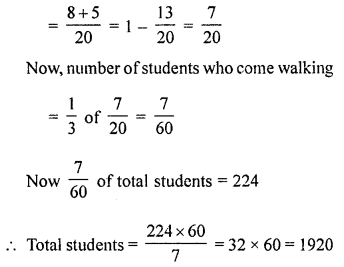Question 14.
A mother and her two sons got a room constructed for ₹ 60,000. The elder son contributes $$\frac { 3 }{ 8 }$$ of his mother’s contribution while the younger son contributes $$\frac { 1 }{ 2 }$$ of his mother’s share. How much do the three contribute individually?
Solution:
Cost of a room = ₹ 60000
Contribution of elder son = $$\frac { 3 }{ 8 }$$ of her mother’s contribution
and contribution of younger son = $$\frac { 1 }{ 2 }$$ of his mother’s share
Let mother contribution = 1
Then elder son’s contribution = $$\frac { 3 }{ 8 }$$
and younger’s = $$\frac { 1 }{ 2 }$$
Now ratios in their share = 1 : $$\frac { 3 }{ 8 }$$ : $$\frac { 1 }{ 2 }$$
= 8 : 3 : 4
Sum of ratios = 8 + 3 + 4 = 15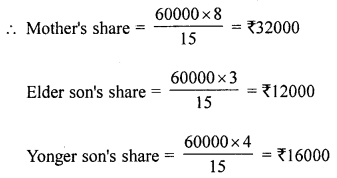Question 15.
In a class of 56 students, the number of boys is $$\frac { 2 }{ 5 }$$ th of the number of girls. Find the number of boys and girls.
Solution:
Number of students in a class = 56
Let number of girls = 1Question 16.
A man donated $$\frac { 1 }{ 10 }$$ of his money to a school, $$\frac { 1 }{ 6 }$$ th of the remaining to a church and the remaining money he distributed equally among his three children. If each child gets ₹ 50000, how much money did the man originally have?
Solution:
Let total money of a man = 1
Amount donated to a school = $$\frac { 1 }{ 10 }$$
Remaining money = 1 – $$\frac { 1 }{ 10 }$$ = $$\frac { 9 }{ 10 }$$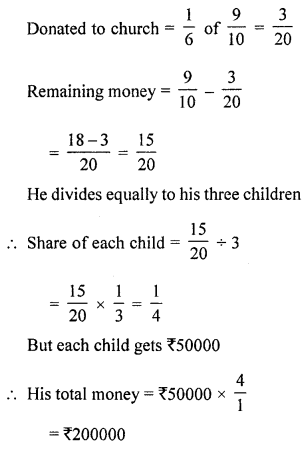Question 17.
If $$\frac { 1 }{ 4 }$$ of a number is added to $$\frac { 1 }{ 3 }$$ of that number, the result is 15 greater than half of that number. Find the number.
Solution:
Let a number be = x
Then according to the condition,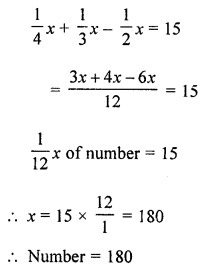Question 18.
A student was asked to multiply a given number by $$\frac { 4 }{ 5 }$$. By mistake, he divided the given number by $$\frac { 4 }{ 5 }$$. His answer was 36 more than the correct answer. What was the given number?
Solution:
Let given number = x
According to the condition,